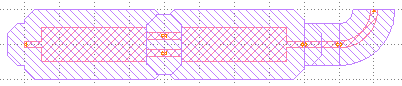Home Forums Nazca Questions and Answers Using “xsections” with a custom building block Reply To: Using “xsections” with a custom building block

#6608

Dear Milan,

A “NoFill” layer to protect from tiling is a specific layer. You can add that to your xsection definition and use interconnects. Whenever you use that interconnect your get the NoFill layer.

In another case, when working directly with Polygon objects, you can grow the Polygon (with pyclipper installed) and redirect the result to the NoFill layer.

The above solutions look like this when applied to the MMI polygon tutorial:

``````import nazca as nd

grow = 5.0  # NoFill clearance

# create a layers and xsections:
nd.add_layer(name='NoFill', layer=2, accuracy=0.1)  # NoFill can be course, here 0.1 um resolution
ic = nd.interconnects.Interconnect(xs="myXS", width=2.0, radius=10.0)  # create interconnect

# create a building block (cell) from Polygon points and add the NoFill layer
with nd.Cell('building_block') as bb:
bb_body = [
(5.0, -5.0), (5.0, -1.0), (0.0, -1.0), (0.0, 1.0),
(5.0, 1.0), (5.0, 5.0), (35.0, 5.0), (35.0, 3.5),
(40.0, 3.5), (40.0, 1.5), (35.0, 1.5), (35.0, -1.5),
(40.0, -1.5), (40.0, -3.5), (35.0, -3.5), (35.0, -5.0)
]
poly = nd.Polygon(points=bb_body, layer='layer1')
poly.put(0)
poly.grow(layer='NoFill', grow=grow).put(0)  # add NoFill layer based on original Polygon.

nd.Pin('a0').put(0, 0, 180)
nd.Pin('b0').put(40, 2.5, 0)
nd.Pin('b1').put(40, -2.5, 0)
nd.put_stub()

#  draw MMIs and interconnects and automatically get NoFill layers:
bb.put(0)
mmi = bb.put('b1')
ic.strt(length=10).put(mmi.pin['a0'])
ic.bend(angle=90).put()

nd.export_gds(filename="nofill")``````A less surgical alternative is to use the cell’s bounding box and fill it with the NoFill layer (not shown in the example above).

Ronald